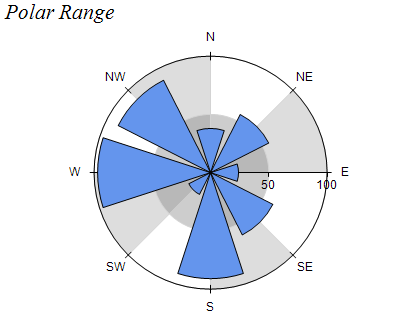﻿ Polar Range
Nevron .NET Vision
Chart for .NET / User's Guide / Chart Types / Polar / Polar Range

In This Topic
Polar Range
In This Topic

A Polar Range chart displays ranges in a polar coordinate system. Each range is specified by its begin and end value and its begin and end angle. The following figure displays a Polar Range chart.Figure 1.

Creating a Polar Range series

Polar Range series are represented by the NPolarRangeSeries type. An instance of this type must be added to the series collection of a Polar Chart (NPolarChart object).

C#
Copy Code
```// clear the Charts collection and create a new polar chart
NPolarChart chart = new NPolarChart();
nChartControl1.Charts.Clear();
// add a polar range series to the chart
NPolarRangeSeries polarRange = new NPolarRangeSeries();
```
Visual Basic
Copy Code
```' clear the Charts collection and create a new polar chart
Dim chart As New NPolarChart
NChartControl1.Charts.Clear()
' add a polar range series to the chart
Dim polarRange As New NPolarRangeSeries
```
Passing Data

Once the series is created you can add some data in it. Polar range series use the Values, Y2Values to specify begin/end values and Angles and X2Values to specify the range begin/end angle. The following code snippet adds a range to a polar range chart:

C#
Copy Code

Visual Basic
Copy Code

Formatting commands

Besides the standard formatting commands you can use the following commands in format strings:

<angle_in_degrees> - the current data point begin angle in degrees (extracted from the Angles data series)
<angle_in_radians> - the current data point begin angle in radians (extracted from the Angles data series)

<angle2_in_degrees> - the current data point end angle in degrees (extracted from the X2Values data series)
<angle2_in_radians> - the current data point end angle in radians (extracted from the X2Values data series)

Related Examples
Windows forms: Chart Gallery\Polar\Polar Range